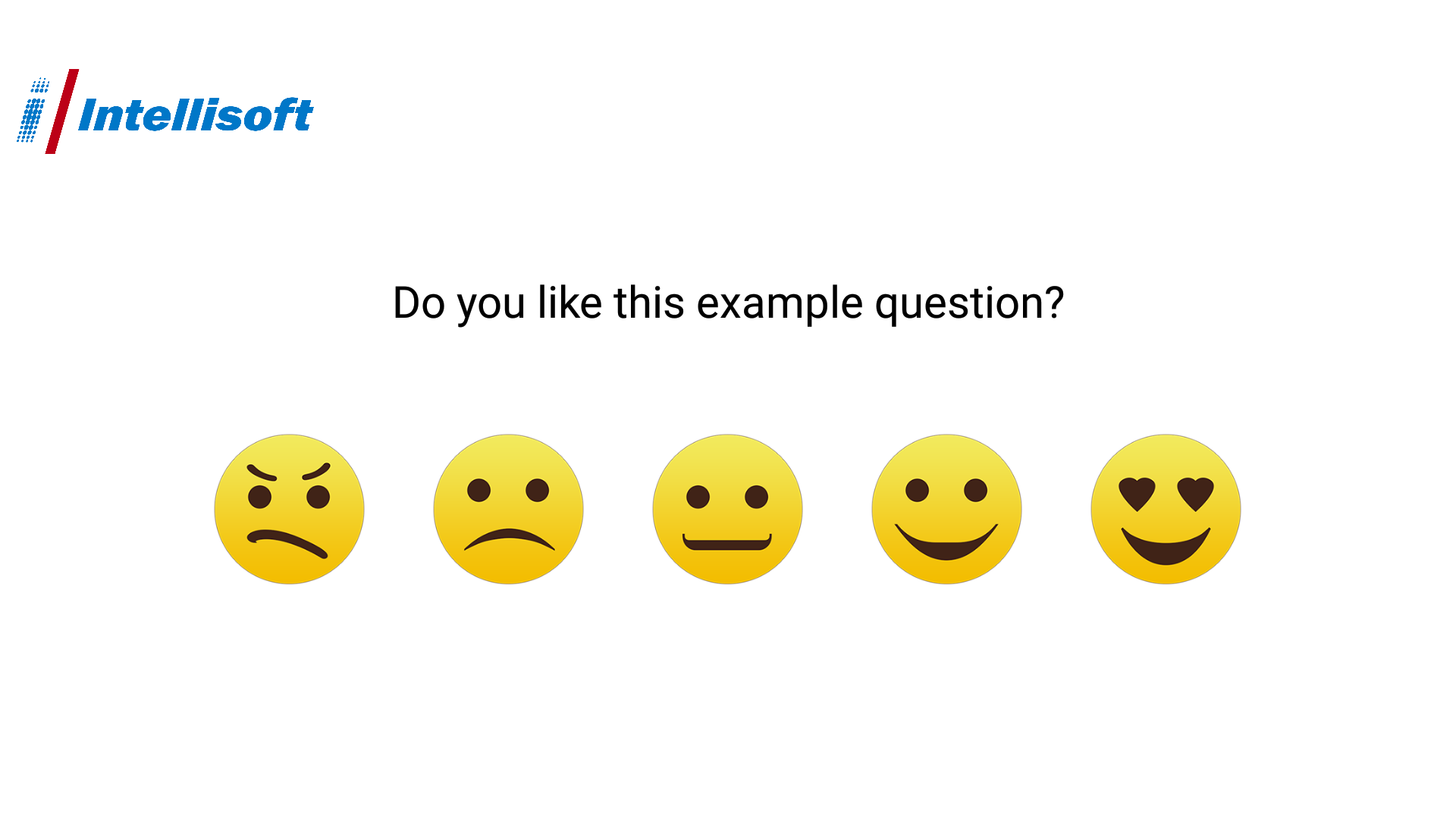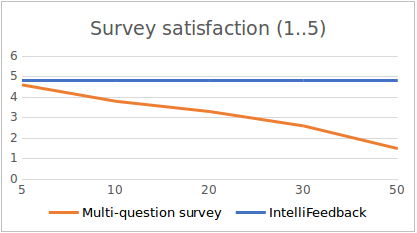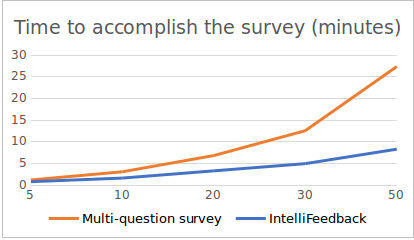### IntelliFeedbackSurvey

Survey list or one question feedback?

It is well known that customer feedback is as important as the way to retrieve it. Therefore we have conducted a research on this on a set of 426 subjects who were asked to answer some questions on tablet PC’s. We took into account:

1. A classic survey, containing up to 50+ questions on one hand. In this case, each subject was supposed to answer each question.
2. IntelliFeedback, which consists of a set of questions, each subject being presented with only one. The combined data from the answers creates a statistically significant result. Figure 1.Figure 1. IntelliFeedback - the one question feedback

Three aspects have been studied in this research, namely: (1) the satisfaction of answering the survey, (2) the time needed to do that and (3) the relevance of the results.

User satisfaction

After the survey, we asked the subjects to assess their satisfaction related to filling in the feedback, on a scale from 1 to 5 (Figure 2). Of course, the satisfaction of filling IntelliFeedback is independent of the number of questions available as one subject answers only one question. On the other hand, the number of questions of a classic multi-question survey influenced negatively the satisfaction, which decreased from 4.6 when the subjects were shown 5 questions to 1.5 when the survey contained 50 questions.Figure 2. The customer satisfaction about accomplishing the survey

Time needed to accomplish the survey

Subsequently we measured the time needed to accomplish the two types of feedback. Figure 3 depicts the time (measured in minutes) for the same subject to answer the number of questions varying from 5 to 50. It is important to notice that even when the same user answered 50 questions with IntelliFeedback, the accomplishing time was significantly reduced than in the case of the classical multi-question surveys.Figure 3. Time to accomplish the surveys

Relevance of the results

While the two indicators (satisfaction and time) refer to the subjects, the third one – relevance of the results – refer to the surveyor. Therefore, the question at stake is how relevant IntelliFeedback is compared to the classic multi-question surveys.

The relevance was dependable on the number of subjects answering a specific question. However, the threshold is quite low, namely 8.4 users answering one question. This means that, if a question is answered by more than 9 people, the result is relevant.

Conclusions

IntelliFeedback is a tool which captures the customer feedback pretty accurately, but without time consuming or annoying questions. However, it is relevant if there are at least 9 people answering a specific question.

Disclaimers

IntelliFeedback is an Android app, adapted for tablets, available in two versions:

Your feedback will be much appreciated. Thank you!